GFG App
Open AppBrowser
Continue

Atom is the smallest unit into which matter can be divided without the release of electrically charged particles. It is also the smallest unit of matter that has the characteristic properties of a chemical element. As such, the atom is the basic building block of matter. Atoms are extremely small, that they can’t be seen through naked eyes or a normal microscope. Most of the space in an atom is empty. The rest consists of a positively charged nucleus of protons and neutrons surrounded by a cloud of negatively charged electrons. The nucleus is small and dense compared with the electrons, which are the lightest charged particles in nature. Electrons are attracted to any positive charge by their electric force. In an atom, electric forces bind the electrons to the nucleus.

Every atom is composed of a nucleus and one or more electrons bound to the nucleus. The nucleus is made of one or more protons and a number of neutrons. More than 99.94 % of an atom’s mass is in the nucleus.

### Composition of an Atom

Because of the nature of quantum mechanics, no single theory has been entirely satisfactory at visualizing the atom’s various characteristics, which thus forces physicists to use complementary pictures of the atom to explain different properties. In some aspects, the electrons in an atom behave like particles orbiting the nucleus. In others, the electrons behave like waves around the nucleus. Such wave patterns are known as orbitals. And these orbitals have the maximum probability to have electrons. The behaviour of an atom is strongly influenced by these orbital properties, and its chemical properties are determined by orbital groupings known as shells.

In simple words, the structure of an atom can be described as similar to our solar planet in which the planet revolves around the sun. As the planet revolves around the sun with the sun as a centre. In a similar fashion, electrons revolve around the nucleus. And as the sun is heavier than all planets of the solar system. In a similar manner, the nucleus contains most of the mass of the atom. But there is a dissimilarity also that sun has sufficient amount of size in our solar system and on the other hand size of the nucleus is almost negligible with respect to atom.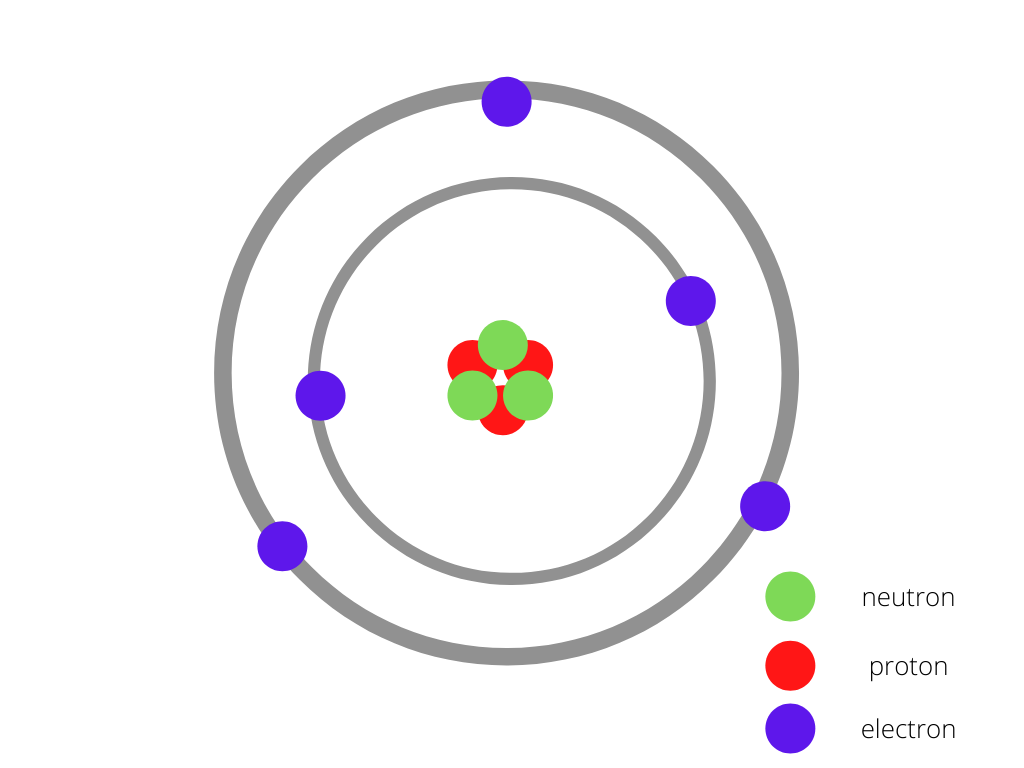Atomic Model

### Variation in the number of Protons, Electrons and Neutrons in different atoms

The atom of each element differs in the number of electrons, protons and neutrons. As we move ahead in the periodic table, the atomic number increases. And similarly the number of protons, neutrons, electrons also increases and accordingly size increases.

Electron: The electron is a subatomic particle, whose electric charge is negative one elementary charge. Electrons belong to the first generation of the lepton particle family and are generally thought to be elementary particles because they have no known components or substructure.

Proton: A proton is a subatomic particle, symbol p or p⁺, with a positive electric charge of +1e elementary charge and a mass slightly less than that of a neutron. Protons and neutrons, each with masses of approximately one atomic mass unit, are jointly referred to as “nucleons”.

Neutron: The neutron is the particle in the atomic nucleus with a mass = 1 and charge = 0. Neutrons are found together with protons in the atomic nucleus. The number of neutrons in an atom determines its isotope.

Although a neutron has a net neutral electrical charge, it does consist of charged components that cancel each other out with respect to charge.

There can be mainly three types of changes in element:

• When the number of protons in an atom is changed, you will change the atom from one element to a different element.
• If you change the number of electrons in an atom, you will get an ion of the element.
• If the numbers of neutrons are changed without changing the number of protons and electrons, then isotopes are formed. The difference in the number of neutrons between the various isotopes of an element means that the various isotopes have different masses.

With the overall combined effect, we can say that atoms of different elements have different numbers of protons, neutrons and electrons.

Lets now consider the following examples to understand the above-mentioned concept:

Example 1:  Carbon (C)

The number of protons in a Carbon atom = 6

The number of electrons in a Carbon atom = 6

The number of neutrons in a Carbon atom = 6

The figure given below shows the structure of the carbon atom has 6 protons and 6 neutrons at the centre surrounded by 6 electrons.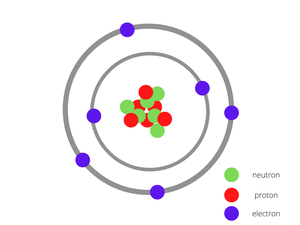Carbon Atom

Example 2: Hydrogen (H)

The number of protons in a Hydrogen atom = 1

The number of electrons in a Hydrogen atom = 1

The number of neutrons in a Hydrogen atom = 0

The figure is given below shows the structure of a hydrogen atom having single protons at the centre and an electron revolving around it.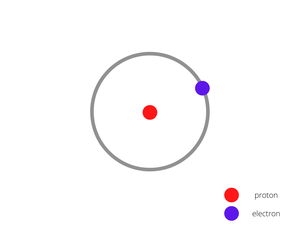Hydrogen Atom

Example 3: Sodium

The number of protons in a Sodium atom = 11

The number of electrons in a Sodium atom = 11

The number of neutrons in a Sodium atom = 12

The figure given below shows the structure of the Sodium atom has 11 protons and 12 neutrons at the centre surrounded by 11 electrons.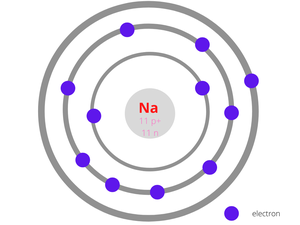Sodium Atom

Example 4: Neon

The number of protons in a Neon atom = 10

The number of electrons in a Neon atom = 10

The number of neutrons in a Neon atom = 10

The figure given below shows the structure of the Neon atom which is an inert gas having 10 protons and 10 neutrons at the centre surrounded by 10 electrons.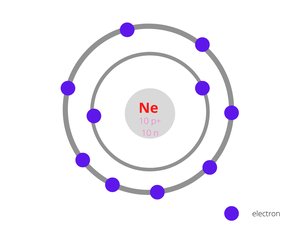Neon Atom

### Sample Questions

Question 1: Calculate the number of protons, electrons, neutrons in Bromine with atomic number 35 and mass number 80.

Step 1: In this step we will find the number of protons of the given sample:

Number of protons of a sample = Atomic Number of the element = 35

Step 2: In this step we will find the number of electrons of the given sample:

Number of electrons of a sample = Number of protons = 35

Step 3: In this step we will find the number of neutrons of the given sample:

Number of neutrons of a sample = (Mass number – Atomic Number) of the element

Number of neutrons of a sample = 80 – 35 = 45

So, here we got our required values.

The values are:

Number of proton, p = 35

Number of neutron, n = 45

Number of electron, e = 35

Question 2: Calculate the number of electrons, protons and neutrons in Titanium (Atomic number = 22, Mass number = 48).

The formula to calculate the number of neutrons is:

Number of neutrons = (A – Z)

where,

A = Mass number

Z = Atomic number

As the atomic number of Titanium is 22 which means the number of protons or electrons in this element is also 22.

Calculation of number of neutrons in Titanium (Ti) as follows:-

Given that Atomic number (Z) of Ti = 22, Mass number (A) of Ti = 48

Number of neutrons = (A – Z)

Number of neutrons in Ti = (48 – 22) = 26

Therefore the number of electrons, protons and neutrons in Titanium are 22, 22 and 26 respectively.

Question 3: Find the number of protons, electrons and neutrons in the nucleus of 13Al27.

Atomic mass (A) = 27

Atomic number (Z) = 13

So, number of  proton =13

Number of neutrons = (A – Z)

neutron = 27−13=14

electron = 13

But, there are only proton and neutron in the nucleus.

So, 13 protons ,14 neutrons and no electrons are present in nucleus of Al .

Question 4: Find the total number of protons, electrons and neutrons in 12 g of 6C12.

6C12 contains 6 N protons, 6NA electrons and 6N neutrons.

Since 1g element contains 1 mole atoms and,

1 mole = 6.023 × 1023

So, Total number of particles =18NA

​=18 × 6.023 × 1023

=1.08 × 1025

So, total number of electrons, protons and neutrons is 1.08 x 1025 .

Question 5: What is the mass number for beryllium with 5 neutrons?

Through the periodic table, by observing in sequence you will find that atomic number of beryllium is 4.

Given , number of neutrons is 5.

Mass number = atomic number + number of neutrons

= 5+4

=  9

So, the mass number for beryllium with 5 neutrons is 9.

My Personal Notes arrow_drop_up